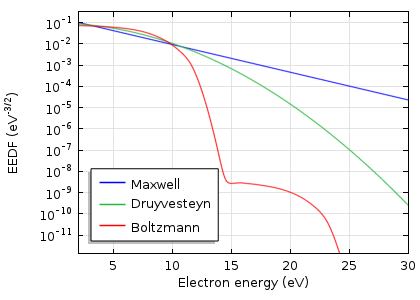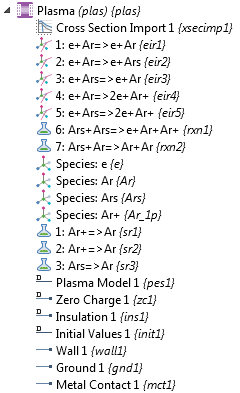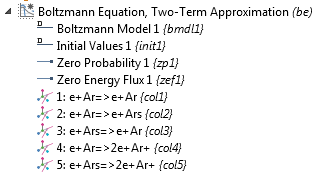# Introduction to Plasma Modeling with Non-Maxwellian EEDFs

July 5, 2017

Plasma modeling normally requires knowing the electron energy distribution function (EEDF) as well as transport properties like electron mobility and diffusivity. To accurately calculate these quantities with the Boltzmann equation, we must also know the electron density (and possibly the density of all species subject to electron impact reactions). However, the electron (and species densities) are outputs of a plasma model, resulting in a catch-22. Let’s take a look at how to overcome this challenge using an example app.

### EEDF and Plasma Modeling

While it’s important to model plasma accurately (to match experimental results, for example), we also want the model to be as simple as possible. To keep the model simple, we typically choose a Maxwellian or Druyvesteyn EEDF and a constant electron mobility. The transport properties can then be computed automatically using the Einstein relation. However, at low-pressure discharges, the EEDF often deviates significantly from a Maxwellian EEDF. At higher pressures, high accelerating fields might also result in a non-Maxwellian distribution.

For a more accurate EEDF shape, we can solve the Boltzmann equation (typically, the two-term approximation). The COMSOL Multiphysics® software even provides a dedicated interface for this purpose — appropriately named the Boltzmann Equation, Two-Term Approximation interface.Comparison of Maxwell, Druyvesteyn, and Boltzmann distribution functions for a typical argon discharge at a mean electron energy of 5 eV.

We then run into a catch-22. To compute the EEDF with the Boltzmann equation, we must have the electron density. This is the result of the plasma model, which we solve using the EEDF and transport properties.

How can we get out of this loop? Well, one possibility is to use an iterative process. The first step is to guess the mean electron density, using it to calculate the EEDF with the Boltzmann Equation, Two-Term Approximation interface. Next, we compute the plasma model with the EEDF to get a new mean electron density. We must then recompute the EEDF, the plasma model, and so on.

Performing this procedure one step at a time can be annoying. A better option is to use the Application Builder to design an app that automates this process. Let’s explore one such example from the Application Gallery.

### Using an App to Model Plasma with a Non-Maxwellian EEDF

To use the app, we only need an initial guess for the electron density and the mole fraction of excited argon atoms. The values for the applied voltage, reactor length, gas temperature, and other variables can be adapted. We can also add a quick circuit at the electrode on the right side. Next, we select our desired type of EEDF (Boltzmann, Maxwellian, Druyvesteyn, or generalized).

When we click the Compute all button, the app automatically alternates between calculating the plasma model and the EEDF model. Each time, the computed mean electron density of the plasma model is used as an input parameter to determine a new EEDF. This continues until the deviation in mean electron density before and after calculating the plasma model falls below a user-defined value. Alternatively, we can solve only the Boltzmann or plasma model by clicking either the Compute Boltzmann only button or the Compute Plasma only button, respectively.

As for our results, we get the plasma species densities, like electron and ion density, as well as the electron temperature. The EEDF and electron transport properties for different mean electron energies are also shown. Using the EEDF at specified position tab under Boltzmann Analysis, we can examine the EEDF at each point in the reactor geometry as well as compare the computed EEDF with a Maxwellian EEDF. In addition, the app evaluates mean values for species densities and mean electron energy.

### The Underlying Plasma and EEDF Models

Let’s take a look at the underlying models. To model plasma with a non-Maxwellian EEDF, we need two models:

1. A plasma model
2. A model to compute the EEDF

The plasma model used in the app is a simple 1D glow discharge model, similar to the one in the Application Gallery. The left electrode of the plasma reactor is grounded, while a voltage is applied to the right. We set the value of this voltage (as well as other parameters like the reactor length, gas pressure, and temperature) in the app.The model tree for the Plasma interface.

We also use a simple 1D model to compute the EEDF. Here, the x-axis does not represent a space coordinate, but rather the electron energy. We then perform a parametric sweep over the mean electron energies. As an example, this model corresponds with the Argon Boltzmann Analysis tutorial.The model tree for the Boltzmann Equation, Two-Term Approximation interface.

It is important to note that this app uses the mean electron density in the reactor to compute the EEDF. So, the EEDF only depends on the average properties of the discharge. In reality, the EEDF should be computed at every point in the reactor geometry. This would be tremendously expensive to compute and is thus avoided in this example.

• Browse these blog posts related to plasma modeling:

#### Catégories##### siva pm
March 4, 2018

How can I know the cross section import data reactions and the format?##### Annette Pahl
March 5, 2018

A lot of cross sections are available online, for example on the LXcat website (https://fr.lxcat.net/home/). More information about the format can be found in the Plasma Module User’s Guide in the section “Importing Collision Cross-Section Data” on pp. 33-36 (version 5.3a).##### Zhumei Sun
May 9, 2018

Is there a cross section data for air? Or I have to generate it from the composition species, oxygen and nitrogen, can comsol do that? I saw the example of corona discharge in air in which A is used as “air molecule”. I would like to know how to get the transport data and cross section for air via bolztmann analysis. Thanks.

EXPLORE COMSOL BLOG